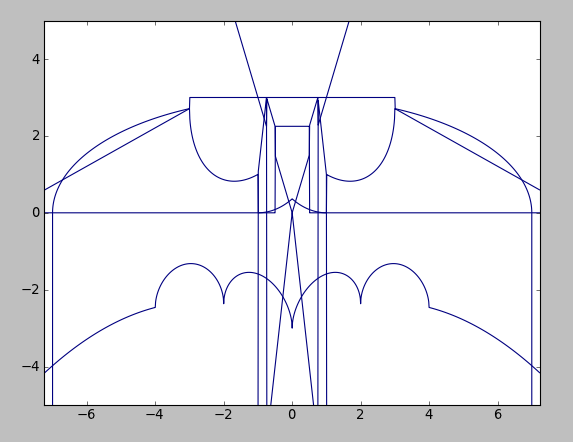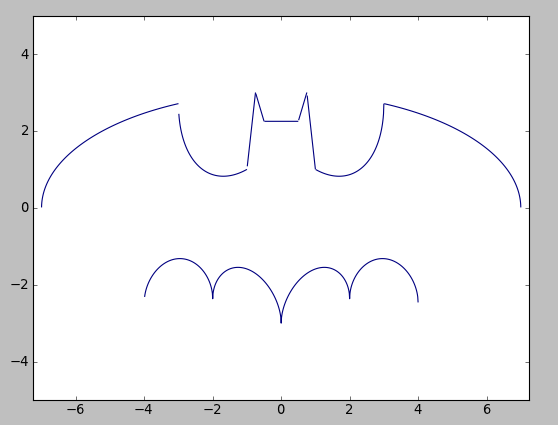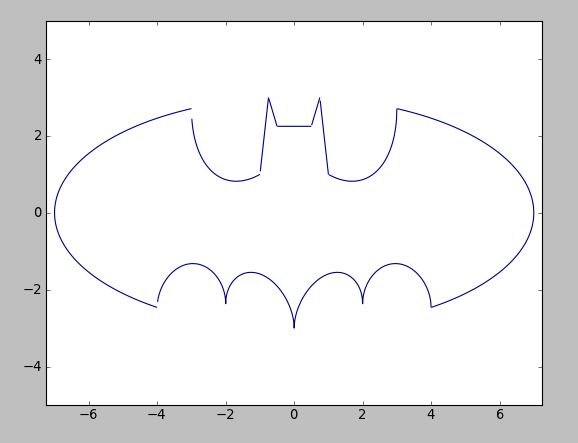# Batman Equation RevisitedI decided to revisit the Batman Equation briefly, but this time at a Python approach, instead of Matlab. The languages are close enough that it shouldn't take very to plug the info in and run...or so I thought.

### Giving it an Initial Go

It started out easy enough. From my Batman Equation Gist I grabbed the 6 equations and pasted them into `batman.py` to run and hope it worked. First thing I had to do was change the syntax for the power function. So a simple Find/Replace of "^" to "**" cleaned that up quickly. I also removed all the semicolons from the end-on-lines.

```import matplotlib.pyplot as plt #To be capable of plotting
from scipy import sqrt
```

I also had to think about how to handle the equations properly. Since Python doesn't have a qualifier like Matlab's `sym x y`, we'll give Python a complete grid of x and y points to work with.

```from numpy import meshgrid
from numpy import arange

xs = arange(-7.25, 7.25, 0.01)
ys = arange(-5, 5, 0.01)
x, y = meshgrid(xs, ys)
```

This should be essentially it. Now we plot:

```for f in [eq1,eq2,eq3,eq4,eq5,eq6]:
plt.contour(x, y, f, )

plt.show()
```WHAT!?

### Problems

#### Complex Numbers

So the first problem I had was the simple one. I was using `sqrt` from scipy which handles complex numbers and returns the real portion. I needed to remain in the real subset of numbers, so I change this line to:

```from numpy import sqrt
```

Success?No...

#### The Hard Part

So it looks close, but the "wings" are missing half the line and it's at 0. So first think I thought of is that the complex-to-real is blowing things up. So I took 'eq1' and tore it apart. Basically it's an ellipse with the center section cut out. So I carved it up into the elements to find the offending piece.

```eq1 = (x/7)**2+(x/3)**2-1 # Plots ellipse beautifully
eq1 = ((x/7)**2*sqrt(abs(abs(x)-3)/(abs(x)-3))+(x/3)**2-1 # Cuts the middle out and looks good
eq1 = ((x/7)**2*sqrt(abs(abs(x)-3)/(abs(x)-3))+(y/3)**2*sqrt(abs(y+3/7*sqrt(33))/(y+3/7*sqrt(33)))-1) # Fails
```

So the offending block `sqrt(abs(y+3/7*sqrt(33))/(y+3/7*sqrt(33)))` is what's causing problems.

Just using the IPython interpreter I laboriously realized that Python was evaluating `3/7` as 0. Which of course we all know is not true. So after a wealth of internet searching and more, I found that I needed to force Python out of floor division for integers. Totally something new to me and can't believe it took me so long to find out. Turns out Python 3 corrects this, but I'm using 2.7.

I first thought I had was to add `.0` to all my integers in my equations, but found that adding this took care of it for me:

```from __future__ import division
```Success!

Grab the Python code here: batman.py